It is currently 17 Nov 2019, 03:17### GMAT Club Daily Prep

#### Thank you for using the timer - this advanced tool can estimate your performance and suggest more practice questions. We have subscribed you to Daily Prep Questions via email.

Customized
for You

we will pick new questions that match your level based on your Timer History

Track

every week, we’ll send you an estimated GMAT score based on your performance

Practice
Pays

we will pick new questions that match your level based on your Timer History

#### Not interested in getting valuable practice questions and articles delivered to your email? No problem, unsubscribe here.# The random variable X is normally distributedQuestion banks Downloads My Bookmarks Reviews Important topics
Author Message
TAGS:Retired ModeratorJoined: 07 Jun 2014
Posts: 4808
GRE 1: Q167 V156WE: Business Development (Energy and Utilities)
Followers: 145

Kudos [?]: 2278  , given: 393

The random variable X is normally distributed [#permalink]
5
KUDOS
Expert's post00:00

Question Stats:36% (00:55) correct63% (00:51) wrongbased on 141 sessions
The random variable X is normally distributed. The values 650 and 850 are at the 60th and 90th percentiles of the distribution of X, respectively.

 Quantity A Quantity B The value at the 75th percentile of the distribution of X 750

A)The quantity in Column A is greater.
B)The quantity in Column B is greater.
C)The two quantities are equal.
D)The relationship cannot be determined from the information given.

Practice Questions
Question: 5
Page: 156
Difficulty: hard
[Reveal] Spoiler: OA

_________________

Sandy
If you found this post useful, please let me know by pressing the Kudos Button

Try our free Online GRE TestRetired ModeratorJoined: 07 Jun 2014
Posts: 4808
GRE 1: Q167 V156WE: Business Development (Energy and Utilities)
Followers: 145

Kudos [?]: 2278  , given: 393

Re: The random variable X is normally distributed [#permalink]
2
KUDOS
Expert's post
Now this is a question that essentially tests our understanding of the bell curve.Now given above is a standard bell curve. When we say value of 60%tile is 650 we mean that it is the area under the bell curve from 0%tile to 60%tile. And
When we say value of 90%tile is 850 we mean that it is the area under the bell curve from 0%tile to 90%tile. Now 75%tile is exactly in the middle between 60%tile and the 90%tile. However there is more area of the bell curve under 60%tile to 75%tile than 75%tile to 90%tile.

Hence the value of 75th %tile should be lower to than 750 (which is half way between 650 and 850).

[Reveal] Spoiler: image
Attachment:Quant.jpg [ 22.35 KiB | Viewed 40119 times ]

_________________

Sandy
If you found this post useful, please let me know by pressing the Kudos Button

Try our free Online GRE TestInternJoined: 14 Jun 2016
Posts: 1
Followers: 0

Kudos [?]: 1  , given: 0

Re: The random variable X is normally distributed [#permalink]
1
KUDOS
Isn't that the other way around?
The value corresponding to the 75th percentile will be closer to the mean and 650 and further from 850. Thus this value will be less that 750 => B
Retired ModeratorJoined: 07 Jun 2014
Posts: 4808
GRE 1: Q167 V156WE: Business Development (Energy and Utilities)
Followers: 145

Kudos [?]: 2278 , given: 393

Re: The random variable X is normally distributed [#permalink]
Expert's post
thanosro wrote:
Isn't that the other way around?
The value corresponding to the 75th percentile will be closer to the mean and 650 and further from 850. Thus this value will be less that 750 => B

Yes you are correct! B is greater.

Thanks for the correction.
_________________

Sandy
If you found this post useful, please let me know by pressing the Kudos Button

Try our free Online GRE TestGRE InstructorJoined: 10 Apr 2015
Posts: 2560
Followers: 91

Kudos [?]: 2725  , given: 40

Re: The random variable X is normally distributed [#permalink]
18
KUDOS
Expert's post
sandy wrote:
The random variable X is normally distributed. The values 650 and 850 are at the 60th and 90th percentiles of the distribution of X, respectively.

 Quantity A Quantity B The value at the 75th percentile of the distribution of X 750

VERY tricky question.

When it comes to normal distributions, it might be useful to think of the area under the curve as the ENTIRE population being examined.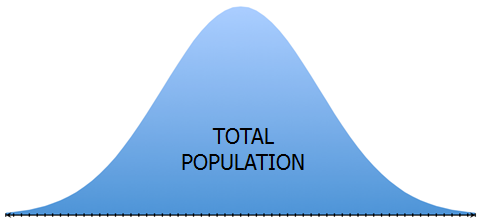In fact, it helps more of you think of each blue pixel/dot under the curve as representing one member of the population.

So, if a score of 650 is at the 60th percentile, we know that 60% of all of the blue pixels/dots lie to the left of 650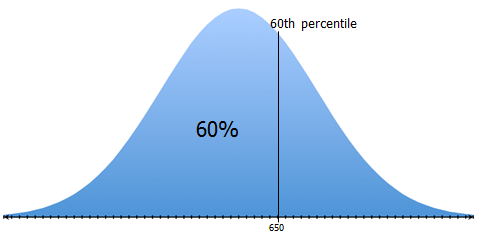Likewise, if a score of 850 is at the 90th percentile, we know that 90% of all of the blue pixels/dots lie to the left of 850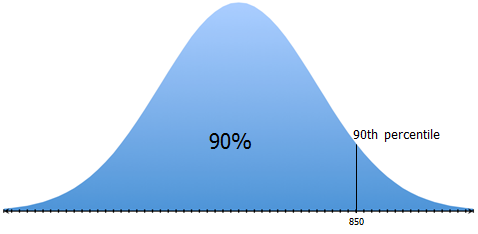IMPORTANT: If we draw a new line that takes the population BETWEEN the 60th and 90th percentiles and divides that population into two EQUAL populations, then that new line will represent the 75th percentile score.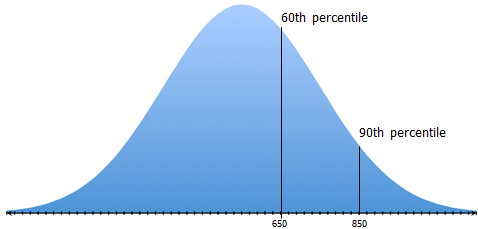So, where should that line go?

Well, if we draw it halfway between 650 and 850...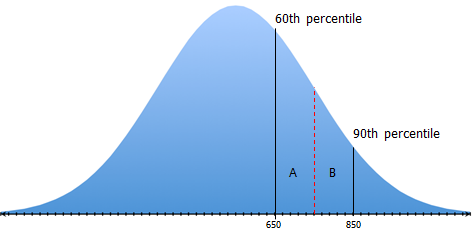...then more of the population will be on side A.
So, this line will NOT divide the population between the 60th and 90th percentiles into two equal populations.

To get a line the DOES divide the population into two equal populations, we need to draw it right about here...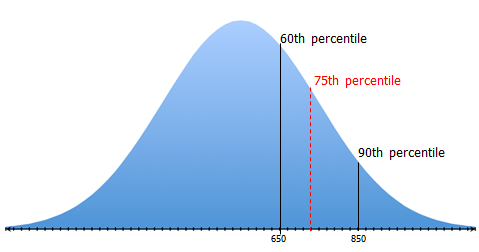Notice that the 75th percentile line is to the left of score of 750 (which is halfway between scores of 650 and 850)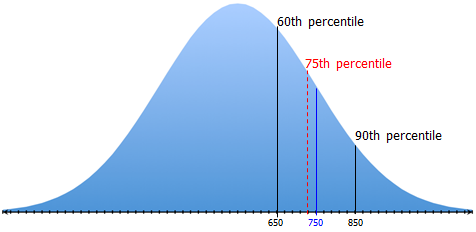So, the SCORE associated with the 75th percentile is LESS THAN 750

_________________

Brent Hanneson – Creator of greenlighttestprep.com

InternJoined: 12 Jul 2017
Posts: 9
Followers: 0

Kudos [?]: 5 , given: 2

Re: The random variable X is normally distributed [#permalink]
Nice explanation with graphics. Clear and clever.! Thanks.ManagerJoined: 27 Feb 2017
Posts: 189
Followers: 1

Kudos [?]: 66  , given: 15

Re: The random variable X is normally distributed [#permalink]
1
KUDOS
I still do not understand how I can solve this type of a question on a graph. I mean visuals are great for practicing and understanding at our own time but in exam, we need to use solid concept and logic for a like aminute or so. I feel like hand drawn thing is a risky guess on exam. So does someone have a better strategy for exam please?Retired ModeratorJoined: 07 Jun 2014
Posts: 4808
GRE 1: Q167 V156WE: Business Development (Energy and Utilities)
Followers: 145

Kudos [?]: 2278  , given: 393

Re: The random variable X is normally distributed [#permalink]
2
KUDOS
Expert's post
kruttikaaggarwal wrote:
I still do not understand how I can solve this type of a question on a graph. I mean visuals are great for practicing and understanding at our own time but in exam, we need to use solid concept and logic for a like aminute or so. I feel like hand drawn thing is a risky guess on exam. So does someone have a better strategy for exam please?

I would argue that Brent is spot on with the best method to solve this problem.

The graph in this case is the logic. In order to uunderstant percentiles and probability distribution you need to understnad what percentile mean on the graph and what a probabaility distribution looks like.

Unfortunately, there is no short cut to solve these problems and these tend to be one of the most difficult problems on the GRE.
_________________

Sandy
If you found this post useful, please let me know by pressing the Kudos Button

Try our free Online GRE TestInternJoined: 27 Aug 2018
Posts: 36
Followers: 0

Kudos [?]: 17  , given: 7

Re: The random variable X is normally distributed [#permalink]
1
KUDOS
Very nice explanationManagerJoined: 01 Nov 2018
Posts: 87
Followers: 0

Kudos [?]: 49  , given: 22

Re: The random variable X is normally distributed [#permalink]
1
KUDOS
Expert's post
GreenlightTestPrep wrote:
sandy wrote:
The random variable X is normally distributed. The values 650 and 850 are at the 60th and 90th percentiles of the distribution of X, respectively.

 Quantity A Quantity B The value at the 75th percentile of the distribution of X 750

VERY tricky question.

When it comes to normal distributions, it might be useful to think of the area under the curve as the ENTIRE population being examined.In fact, it helps more of you think of each blue pixel/dot under the curve as representing one member of the population.

The halfway point between scores is flat, and can be equal, but if you drew a line from the 750 score to its percentile, it would have to be less than halfway between 650 and 850, since the curve curves downward, with less area.

So, if a score of 650 is at the 60th percentile, we know that 60% of all of the blue pixels/dots lie to the left of 650Likewise, if a score of 850 is at the 90th percentile, we know that 90% of all of the blue pixels/dots lie to the left of 850IMPORTANT: If we draw a new line that takes the population BETWEEN the 60th and 90th percentiles and divides that population into two EQUAL populations, then that new line will represent the 75th percentile score.So, where should that line go?

Well, if we draw it halfway between 650 and 850......then more of the population will be on side A.
So, this line will NOT divide the population between the 60th and 90th percentiles into two equal populations.

To get a line the DOES divide the population into two equal populations, we need to draw it right about here...Notice that the 75th percentile line is to the left of score of 750 (which is halfway between scores of 650 and 850)So, the SCORE associated with the 75th percentile is LESS THAN 750

FounderJoined: 18 Apr 2015
Posts: 8760
Followers: 175

Kudos [?]: 2035 , given: 8089

Re: The random variable X is normally distributed [#permalink]
Expert's post
GreenlightTestPrep wrote:
sandy wrote:
The random variable X is normally distributed. The values 650 and 850 are at the 60th and 90th percentiles of the distribution of X, respectively.

 Quantity A Quantity B The value at the 75th percentile of the distribution of X 750

VERY tricky question.

When it comes to normal distributions, it might be useful to think of the area under the curve as the ENTIRE population being examined.In fact, it helps more of you think of each blue pixel/dot under the curve as representing one member of the population.

So, if a score of 650 is at the 60th percentile, we know that 60% of all of the blue pixels/dots lie to the left of 650Likewise, if a score of 850 is at the 90th percentile, we know that 90% of all of the blue pixels/dots lie to the left of 850IMPORTANT: If we draw a new line that takes the population BETWEEN the 60th and 90th percentiles and divides that population into two EQUAL populations, then that new line will represent the 75th percentile score.So, where should that line go?

Well, if we draw it halfway between 650 and 850......then more of the population will be on side A.
So, this line will NOT divide the population between the 60th and 90th percentiles into two equal populations.

To get a line the DOES divide the population into two equal populations, we need to draw it right about here...Notice that the 75th percentile line is to the left of score of 750 (which is halfway between scores of 650 and 850)So, the SCORE associated with the 75th percentile is LESS THAN 750

Stunning. really...................
_________________

Need Practice? 20 Free GRE Quant Tests available for free with 20 Kudos
GRE Prep Club Members of the Month: Each member of the month will get three months free access of GRE Prep Club tests.Re: The random variable X is normally distributed   [#permalink] 02 Jun 2019, 11:45
Display posts from previous: Sort by

# The random variable X is normally distributedQuestion banks Downloads My Bookmarks Reviews Important topicsPowered by phpBB © phpBB Group Kindly note that the GRE® test is a registered trademark of the Educational Testing Service®, and this site has neither been reviewed nor endorsed by ETS®.